## 2.3 Algebra of Vectors

### Learning Objectives

By the end of this section, you will be able to:

• Apply analytical methods of vector algebra to find resultant vectors and to solve vector equations for unknown vectors.
• Interpret physical situations in terms of vector expressions.

Vectors can be added together and multiplied by scalars. Vector addition is associative ((Figure)) and commutative ((Figure)), and vector multiplication by a sum of scalars is distributive ((Figure)). Also, scalar multiplication by a sum of vectors is distributive:

$\alpha (\overset{\to }{A}+\overset{\to }{B})=\alpha \overset{\to }{A}+\alpha \overset{\to }{B}.$

In this equation, $\alpha$ is any number (a scalar). For example, a vector antiparallel to vector $\overset{\to }{A}={A}_{x}\hat{i}+{A}_{y}\hat{j}+{A}_{z}\hat{k}$ can be expressed simply by multiplying $\overset{\to }{A}$ by the scalar $\alpha =-1$:

$\text{−}\overset{\to }{A}=\text{−}{A}_{x}\hat{i}-{A}_{y}\hat{j}-{A}_{z}\hat{k}.$

### Example

#### Direction of Motion

In a Cartesian coordinate system where $\hat{i}$ denotes geographic east, $\hat{j}$ denotes geographic north, and $\hat{k}$ denotes altitude above sea level, a military convoy advances its position through unknown territory with velocity $\overset{\to }{v}=(4.0\hat{i}+3.0\hat{j}+0.1\hat{k})\text{km}\text{/}\text{h}$. If the convoy had to retreat, in what geographic direction would it be moving?

#### Solution

The generalization of the number zero to vector algebra is called the null vector, denoted by $\overset{\to }{0}$. All components of the null vector are zero, $\overset{\to }{0}=0\hat{i}+0\hat{j}+0\hat{k}$, so the null vector has no length and no direction.

Two vectors $\overset{\to }{A}$ and $\overset{\to }{B}$ are equal vectors if and only if their difference is the null vector:

$\overset{\to }{0}=\overset{\to }{A}-\overset{\to }{B}=({A}_{x}\hat{i}+{A}_{y}\hat{j}+{A}_{z}\hat{k})-({B}_{x}\hat{i}+{B}_{y}\hat{j}+{B}_{z}\hat{k})=({A}_{x}-{B}_{x})\hat{i}+({A}_{y}-{B}_{y})\hat{j}+({A}_{z}-{B}_{z})\hat{k}.$

This vector equation means we must have simultaneously ${A}_{x}-{B}_{x}=0$, ${A}_{y}-{B}_{y}=0$, and ${A}_{z}-{B}_{z}=0$. Hence, we can write $\overset{\to }{A}=\overset{\to }{B}$ if and only if the corresponding components of vectors $\overset{\to }{A}$ and $\overset{\to }{B}$ are equal:

$\overset{\to }{A}=\overset{\to }{B}\enspace⇔\enspace\{\begin{array}{c}{A}_{x}={B}_{x}\\ {A}_{y}={B}_{y}\\ {A}_{z}={B}_{z}\end{array}.$

Two vectors are equal when their corresponding scalar components are equal.

Resolving vectors into their scalar components (i.e., finding their scalar components) and expressing them analytically in vector component form (given by (Figure)) allows us to use vector algebra to find sums or differences of many vectors analytically (i.e., without using graphical methods). For example, to find the resultant of two vectors $\overset{\to }{A}$ and $\overset{\to }{B}$, we simply add them component by component, as follows:

$\overset{\to }{R}=\overset{\to }{A}+\overset{\to }{B}=({A}_{x}\hat{i}+{A}_{y}\hat{j}+{A}_{z}\hat{k})+({B}_{x}\hat{i}+{B}_{y}\hat{j}+{B}_{z}\hat{k})=({A}_{x}+{B}_{x})\hat{i}+({A}_{y}+{B}_{y})\hat{j}+({A}_{z}+{B}_{z})\hat{k}.$

In this way, using (Figure), scalar components of the resultant vector $\overset{\to }{R}={R}_{x}\hat{i}+{R}_{y}\hat{j}+{R}_{z}\hat{k}$ are the sums of corresponding scalar components of vectors $\overset{\to }{A}$ and $\overset{\to }{B}$:

$\{\begin{array}{c}{R}_{x}={A}_{x}+{B}_{x},\\ {R}_{y}={A}_{y}+{B}_{y},\\ {R}_{z}={A}_{z}+{B}_{z}.\end{array}$

Analytical methods can be used to find components of a resultant of many vectors. For example, if we are to sum up $N$ vectors ${\overset{\to }{F}}_{1},{\overset{\to }{F}}_{2},{\overset{\to }{F}}_{3},\dots ,{\overset{\to }{F}}_{N}$, where each vector is ${\overset{\to }{F}}_{k}={F}_{kx}\hat{i}+{F}_{ky}\hat{j}+{F}_{kz}\hat{k}$, the resultant vector ${\overset{\to }{F}}_{R}$ is

$\begin{array}{cc}\hfill {\overset{\to }{F}}_{R}={\overset{\to }{F}}_{1}+{\overset{\to }{F}}_{2}+{\overset{\to }{F}}_{3}+\text{…}+{\overset{\to }{F}}_{N}& =\sum _{k=1}^{N}{\overset{\to }{F}}_{k}=\sum _{k=1}^{N}({F}_{kx}\hat{i}+{F}_{ky}\hat{j}+{F}_{kz}\hat{k})\hfill \\ & =(\sum _{k=1}^{N}{F}_{kx})\hat{i}+(\sum _{k=1}^{N}{F}_{ky})\hat{j}+(\sum _{k=1}^{N}{F}_{kz})\hat{k}.\hfill \end{array}$

Therefore, scalar components of the resultant vector are

$\{\begin{array}{c}{F}_{Rx}=\sum _{k=1}^{N}{F}_{kx}={F}_{1x}+{F}_{2x}+\text{…}+{F}_{Nx}\\ {F}_{Ry}=\sum _{k=1}^{N}{F}_{ky}={F}_{1y}+{F}_{2y}+\text{…}+{F}_{Ny}\\ {F}_{Rz}=\sum _{k=1}^{N}{F}_{kz}={F}_{1z}+{F}_{2z}+\text{…}+{F}_{Nz}.\end{array}$

Having found the scalar components, we can write the resultant in vector component form:

${\overset{\to }{F}}_{R}={F}_{Rx}\hat{i}+{F}_{Ry}\hat{j}+{F}_{Rz}\hat{k}.$

Analytical methods for finding the resultant and, in general, for solving vector equations are very important in physics because many physical quantities are vectors. For example, we use this method in kinematics to find resultant displacement vectors and resultant velocity vectors, in mechanics to find resultant force vectors and the resultants of many derived vector quantities, and in electricity and magnetism to find resultant electric or magnetic vector fields.

### Example

#### Analytical Computation of a Resultant

Three displacement vectors $\overset{\to }{A}$, $\overset{\to }{B}$, and $\overset{\to }{C}$ in a plane ((Figure)) are specified by their magnitudes A = 10.0, B = 7.0, and C = 8.0, respectively, and by their respective direction angles with the horizontal direction $\alpha =35\text{°},$ $\beta =-110\text{°}$, and $\gamma =30\text{°}$. The physical units of the magnitudes are centimeters. Resolve the vectors to their scalar components and find the following vector sums: (a) $\overset{\to }{R}=\overset{\to }{A}+\overset{\to }{B}+\overset{\to }{C}$, (b) $\overset{\to }{D}=\overset{\to }{A}-\overset{\to }{B}$, and (c) $\overset{\to }{S}=\overset{\to }{A}-3\overset{\to }{B}+\overset{\to }{C}$.

#### Strategy

First, we use (Figure) to find the scalar components of each vector and then we express each vector in its vector component form given by (Figure). Then, we use analytical methods of vector algebra to find the resultants.

#### Significance

Having found the vector components, we can illustrate the vectors by graphing or we can compute magnitudes and direction angles, as shown in (Figure). Results for the magnitudes in (b) and (c) can be compared with results for the same problems obtained with the graphical method, shown in (Figure) and (Figure). Notice that the analytical method produces exact results and its accuracy is not limited by the resolution of a ruler or a protractor, as it was with the graphical method used in (Figure) for finding this same resultant.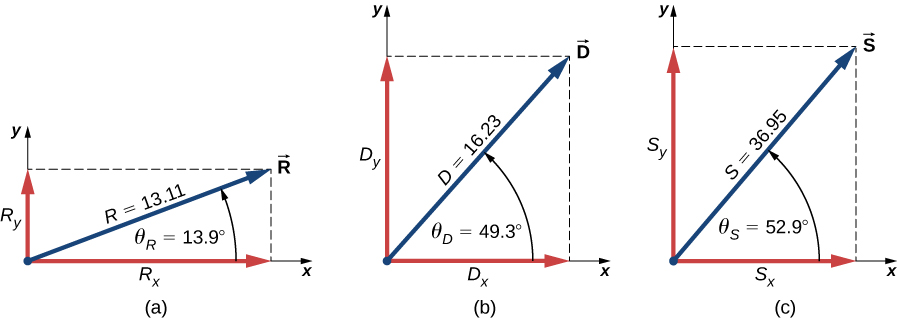Figure 2.24 Graphical illustration of the solutions obtained analytically in (Figure).

Three displacement vectors $\overset{\to }{A}$, $\overset{\to }{B}$, and $\overset{\to }{F}$ ((Figure)) are specified by their magnitudes A = 10.00, B = 7.00, and F = 20.00, respectively, and by their respective direction angles with the horizontal direction $\alpha =35\text{°}$, $\beta =-110\text{°}$, and $\phi =110\text{°}$. The physical units of the magnitudes are centimeters. Use the analytical method to find vector $\overset{\to }{G}=\overset{\to }{A}+2\overset{\to }{B}-\overset{\to }{F}$. Verify that

G = 28.15 cm and that ${\theta }_{G}=-68.65\text{°}$.

$\overset{\to }{G}=(10.25\hat{i}-26.22\hat{j})\text{cm}$

### Example

#### The Tug-of-War Game

Four dogs named Ang, Bing, Chang, and Dong play a tug-of-war game with a toy ((Figure)). Ang pulls on the toy in direction $\alpha =55\text{°}$ south of east, Bing pulls in direction $\beta =60\text{°}$ east of north, and Chang pulls in direction $\gamma =55\text{°}$ west of north. Ang pulls strongly with 160.0 units of force (N), which we abbreviate as A = 160.0 N. Bing pulls even stronger than Ang with a force of magnitude B = 200.0 N, and Chang pulls with a force of magnitude C = 140.0 N. When Dong pulls on the toy in such a way that his force balances out the resultant of the other three forces, the toy does not move in any direction. With how big a force and in what direction must Dong pull on the toy for this to happen?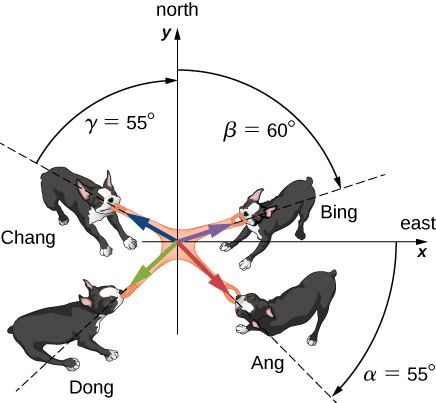Figure 2.25 Four dogs play a tug-of-war game with a toy.

#### Strategy

We assume that east is the direction of the positive x-axis and north is the direction of the positive y-axis. As in (Figure), we have to resolve the three given forces— $\overset{\to }{A}$ (the pull from Ang), $\overset{\to }{B}$ (the pull from Bing), and $\overset{\to }{C}$ (the pull from Chang)—into their scalar components and then find the scalar components of the resultant vector $\overset{\to }{R}=\overset{\to }{A}+\overset{\to }{B}+\overset{\to }{C}$. When the pulling force $\overset{\to }{D}$ from Dong balances out this resultant, the sum of $\overset{\to }{D}$ and $\overset{\to }{R}$ must give the null vector $\overset{\to }{D}+\overset{\to }{R}=\overset{\to }{0}$. This means that $\overset{\to }{D}=\text{−}\overset{\to }{R}$, so the pull from Dong must be antiparallel to $\overset{\to }{R}$.

#### Solution

Suppose that Bing in (Figure) leaves the game to attend to more important matters, but Ang, Chang, and Dong continue playing. Ang and Chang’s pull on the toy does not change, but Dong runs around and bites on the toy in a different place. With how big a force and in what direction must Dong pull on the toy now to balance out the combined pulls from Chang and Ang? Illustrate this situation by drawing a vector diagram indicating all forces involved.

### Example

#### Vector Algebra

Find the magnitude of the vector $\overset{\to }{C}$ that satisfies the equation $2\overset{\to }{A}-6\overset{\to }{B}+3\overset{\to }{C}=2\hat{j}$, where $\overset{\to }{A}=\hat{i}-2\hat{k}$ and $\overset{\to }{B}=\text{−}\hat{j}+\hat{k}\text{/}2$.

#### Strategy

We first solve the given equation for the unknown vector $\overset{\to }{C}$. Then we substitute $\overset{\to }{A}$ and $\overset{\to }{B}$; group the terms along each of the three directions $\hat{i}$, $\hat{j}$, and $\hat{k}$; and identify the scalar components ${C}_{x}$, ${C}_{y}$, and ${C}_{z}$. Finally, we substitute into (Figure) to find magnitude C.

#### Solution

$C=\sqrt{{C}_{x}^{2}+{C}_{y}^{2}+{C}_{z}^{2}}=\sqrt{{(-2\text{/}3)}^{2}+{(\text{−}4\,\text{/}3)}^{2}+{(7\,\text{/}3)}^{2}}=\sqrt{23\text{/}3}.$

### Example

#### Displacement of a Skier

Starting at a ski lodge, a cross-country skier goes 5.0 km north, then 3.0 km west, and finally 4.0 km southwest before taking a rest. Find his total displacement vector relative to the lodge when he is at the rest point. How far and in what direction must he ski from the rest point to return directly to the lodge?

#### Strategy

We assume a rectangular coordinate system with the origin at the ski lodge and with the unit vector $\hat{i}$ pointing east and the unit vector $\hat{j}$ pointing north. There are three displacements: ${\overset{\to }{D}}_{1}$, ${\overset{\to }{D}}_{2}$, and ${\overset{\to }{D}}_{3}$. We identify their magnitudes as ${D}_{1}=5.0\,\text{km}$, ${D}_{2}=3.0\,\text{km}$, and ${D}_{3}=4.0\,\text{km}$. We identify their directions are the angles ${\theta }_{1}=90\text{°}$, ${\theta }_{2}=180\text{°}$, and ${\theta }_{3}=180\text{°}+45\text{°}=225\text{°}$. We resolve each displacement vector to its scalar components and substitute the components into (Figure) to obtain the scalar components of the resultant displacement $\overset{\to }{D}$ from the lodge to the rest point. On the way back from the rest point to the lodge, the displacement is $\overset{\to }{B}=\text{−}\overset{\to }{D}$. Finally, we find the magnitude and direction of $\overset{\to }{B}$.

#### Significance

Notice that no figure is needed to solve this problem by the analytical method. Figures are required when using a graphical method; however, we can check if our solution makes sense by sketching it, which is a useful final step in solving any vector problem.

### Example

#### Displacement of a Jogger

A jogger runs up a flight of 200 identical steps to the top of a hill and then runs along the top of the hill 50.0 m before he stops at a drinking fountain ((Figure)). His displacement vector from point A at the bottom of the steps to point B at the fountain is ${\overset{\to }{D}}_{AB}=(-90.0\hat{i}+30.0\hat{j})\text{m}$. What is the height and width of each step in the flight? What is the actual distance the jogger covers? If he makes a loop and returns to point A, what is his net displacement vector?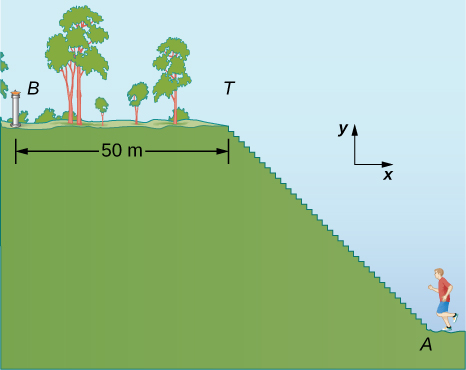Figure 2.26 A jogger runs up a flight of steps.

#### Strategy

The displacement vector ${\overset{\to }{D}}_{AB}$ is the vector sum of the jogger’s displacement vector ${\overset{\to }{D}}_{AT}$ along the stairs (from point A at the bottom of the stairs to point T at the top of the stairs) and his displacement vector ${\overset{\to }{D}}_{TB}$ on the top of the hill (from point T at the top of the stairs to the fountain at point B). We must find the horizontal and the vertical components of ${\overset{\to }{D}}_{TB}$. If each step has width w and height h, the horizontal component of ${\overset{\to }{D}}_{TB}$ must have a length of 200w and the vertical component must have a length of 200h. The actual distance the jogger covers is the sum of the distance he runs up the stairs and the distance of 50.0 m that he runs along the top of the hill.

#### Solution

((Figure)).

In many physical situations, we often need to know the direction of a vector. For example, we may want to know the direction of a magnetic field vector at some point or the direction of motion of an object. We have already said direction is given by a unit vector, which is a dimensionless entity—that is, it has no physical units associated with it. When the vector in question lies along one of the axes in a Cartesian system of coordinates, the answer is simple, because then its unit vector of direction is either parallel or antiparallel to the direction of the unit vector of an axis. For example, the direction of vector $\overset{\to }{d}=-5\,\text{m}\hat{i}$ is unit vector $\hat{d}=\text{−}\hat{i}$. The general rule of finding the unit vector $\hat{V}$ of direction for any vector $\overset{\to }{V}$ is to divide it by its magnitude V:

$\hat{V}=\frac{\overset{\to }{V}}{V}.$

We see from this expression that the unit vector of direction is indeed dimensionless because the numerator and the denominator in (Figure) have the same physical unit. In this way, (Figure) allows us to express the unit vector of direction in terms of unit vectors of the axes. The following example illustrates this principle.

### Example

#### The Unit Vector of Direction

If the velocity vector of the military convoy in (Figure) is $\overset{\to }{v}=(4.000\hat{i}+3.000\hat{j}+0.100\hat{k})\text{km}\text{/}\text{h}$, what is the unit vector of its direction of motion?

#### Strategy

The unit vector of the convoy’s direction of motion is the unit vector $\hat{v}$ that is parallel to the velocity vector. The unit vector is obtained by dividing a vector by its magnitude, in accordance with (Figure).

#### Significance

Note that when using the analytical method with a calculator, it is advisable to carry out your calculations to at least three decimal places and then round off the final answer to the required number of significant figures, which is the way we performed calculations in this example. If you round off your partial answer too early, you risk your final answer having a huge numerical error, and it may be far off from the exact answer or from a value measured in an experiment.

Verify that vector $\hat{v}$ obtained in (Figure) is indeed a unit vector by computing its magnitude. If the convoy in (Figure) was moving across a desert flatland—that is, if the third component of its velocity was zero—what is the unit vector of its direction of motion? Which geographic direction does it represent?

### Summary

• Analytical methods of vector algebra allow us to find resultants of sums or differences of vectors without having to draw them. Analytical methods of vector addition are exact, contrary to graphical methods, which are approximate.
• Analytical methods of vector algebra are used routinely in mechanics, electricity, and magnetism. They are important mathematical tools of physics.

### Problems

For vectors $\overset{\to }{B}=\text{−}\hat{i}-4\hat{j}$ and $\overset{\to }{A}=-3\hat{i}-2\hat{j}$, calculate (a) $\overset{\to }{A}+\overset{\to }{B}$ and its magnitude and direction angle, and (b) $\overset{\to }{A}-\overset{\to }{B}$ and its magnitude and direction angle.

A particle undergoes three consecutive displacements given by vectors ${\overset{\to }{D}}_{1}=(3.0\hat{i}-4.0\hat{j}-2.0\hat{k})\text{mm}$, ${\overset{\to }{D}}_{2}=(1.0\hat{i}-7.0\hat{j}+4.0\hat{k})\text{mm}$, and ${\overset{\to }{D}}_{3}=(-7.0\hat{i}+4.0\hat{j}+1.0\hat{k})\text{mm}$. (a) Find the resultant displacement vector of the particle. (b) What is the magnitude of the resultant displacement? (c) If all displacements were along one line, how far would the particle travel?

Given two displacement vectors $\overset{\to }{A}=(3.00\hat{i}-4.00\hat{j}+4.00\hat{k})\text{m}$ and $\overset{\to }{B}=(2.00\hat{i}+3.00\hat{j}-7.00\hat{k})\text{m}$, find the displacements and their magnitudes for (a) $\overset{\to }{C}=\overset{\to }{A}+\overset{\to }{B}$ and (b) $\overset{\to }{D}=2\overset{\to }{A}-\overset{\to }{B}$.

A small plane flies $40.0\,\text{km}$ in a direction $60\text{°}$ north of east and then flies $30.0\,\text{km}$ in a direction $15\text{°}$ north of east. Use the analytical method to find the total distance the plane covers from the starting point, and the geographic direction of its displacement vector. What is its displacement vector?

In an attempt to escape a desert island, a castaway builds a raft and sets out to sea. The wind shifts a great deal during the day, and she is blown along the following straight lines: 2.50 km and $45.0\text{°}$ north of west, then 4.70 km and $60.0\text{°}$ south of east, then 1.30 km and $25.0\text{°}$ south of west, then 5.10 km due east, then 1.70 km and $5.00\text{°}$ east of north, then 7.20 km and $55.0\text{°}$ south of west, and finally 2.80 km and $10.0\text{°}$ north of east. Use the analytical method to find the resultant vector of all her displacement vectors. What is its magnitude and direction?

Assuming the +x-axis is horizontal to the right for the vectors given in the following figure, use the analytical method to find the following resultants: (a) $\overset{\to }{A}+\overset{\to }{B},$ (b) $\overset{\to }{C}+\overset{\to }{B}$, (c) $\overset{\to }{D}+\overset{\to }{F}$, (d) $\overset{\to }{A}-\overset{\to }{B}$, (e) $\overset{\to }{D}-\overset{\to }{F}$, (f) $\overset{\to }{A}+2\overset{\to }{F}$, (g) $\overset{\to }{C}-2\overset{\to }{D}+3\overset{\to }{F}$, and (h) $\overset{\to }{A}-4\overset{\to }{D}+2\overset{\to }{F}$.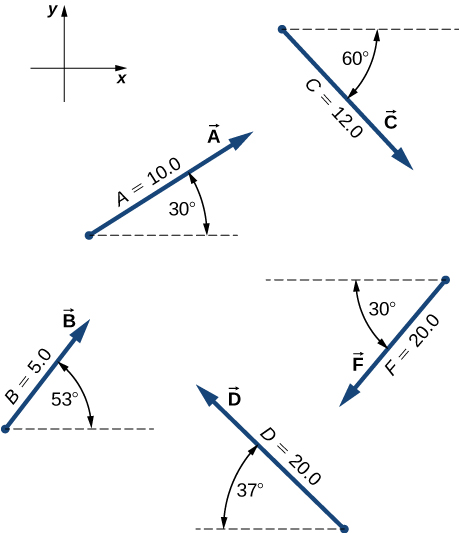Given the vectors in the preceding figure, find vector $\overset{\to }{R}$ that solves equations (a) $\overset{\to }{D}+\overset{\to }{R}=\overset{\to }{F}$ and (b) $\overset{\to }{C}-2\overset{\to }{D}+5\overset{\to }{R}=3\overset{\to }{F}$. Assume the +x-axis is horizontal to the right.

A delivery man starts at the post office, drives 40 km north, then 20 km west, then 60 km northeast, and finally 50 km north to stop for lunch. Use the analytical method to determine the following: (a) Find his net displacement vector. (b) How far is the restaurant from the post office? (c) If he returns directly from the restaurant to the post office, what is his displacement vector on the return trip? (d) What is his compass heading on the return trip? Assume the +x-axis is to the east.

An adventurous dog strays from home, runs three blocks east, two blocks north, and one block east, one block north, and two blocks west. Assuming that each block is about a 100 yd, use the analytical method to find the dog’s net displacement vector, its magnitude, and its direction. Assume the +x-axis is to the east. How would your answer be affected if each block was about 100 m?

If $\overset{\to }{D}=(6.00\hat{i}-8.00\hat{j})\text{m}$, $\overset{\to }{B}=(-8.00\hat{i}+3.00\hat{j})\text{m}$, and $\overset{\to }{A}=(26.0\hat{i}+19.0\hat{j})\text{m}$, find the unknown constants a and b such that $a\overset{\to }{D}+b\overset{\to }{B}+\overset{\to }{A}=\overset{\to }{0}$.

Given the displacement vector $\overset{\to }{D}=(3\hat{i}-4\hat{j})\text{m,}$ find the displacement vector $\overset{\to }{R}$ so that $\overset{\to }{D}+\overset{\to }{R}=-4D\hat{j}$.

Find the unit vector of direction for the following vector quantities: (a) Force $\overset{\to }{F}=(3.0\hat{i}-2.0\hat{j})\text{N}$, (b) displacement $\overset{\to }{D}=(-3.0\hat{i}-4.0\hat{j})\text{m}$, and (c) velocity $\overset{\to }{v}=(-5.00\hat{i}+4.00\hat{j})\text{m/s}$.

At one point in space, the direction of the electric field vector is given in the Cartesian system by the unit vector $\hat{E}=1\,\text{/}\sqrt{5}\hat{i}-2\,\text{/}\sqrt{5}\hat{j}$. If the magnitude of the electric field vector is E = 400.0 V/m, what are the scalar components ${E}_{x}$, ${E}_{y}$, and ${E}_{z}$ of the electric field vector $\overset{\to }{E}$ at this point? What is the direction angle ${\theta }_{E}$ of the electric field vector at this point?

A barge is pulled by the two tugboats shown in the following figure. One tugboat pulls on the barge with a force of magnitude 4000 units of force at $15\text{°}$ above the line AB (see the figure and the other tugboat pulls on the barge with a force of magnitude 5000 units of force at $12\text{°}$ below the line AB. Resolve the pulling forces to their scalar components and find the components of the resultant force pulling on the barge. What is the magnitude of the resultant pull? What is its direction relative to the line AB?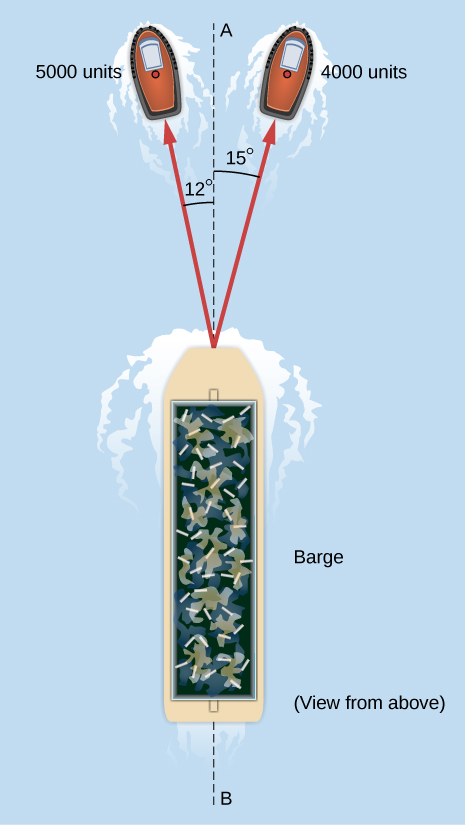In the control tower at a regional airport, an air traffic controller monitors two aircraft as their positions change with respect to the control tower. One plane is a cargo carrier Boeing 747 and the other plane is a Douglas DC-3. The Boeing is at an altitude of 2500 m, climbing at $10\text{°}$ above the horizontal, and moving $30\text{°}$ north of west. The DC-3 is at an altitude of 3000 m, climbing at $5\text{°}$ above the horizontal, and cruising directly west. (a) Find the position vectors of the planes relative to the control tower. (b) What is the distance between the planes at the moment the air traffic controller makes a note about their positions?

### Glossary

equal vectors
two vectors are equal if and only if all their corresponding components are equal; alternately, two parallel vectors of equal magnitudes
null vector
a vector with all its components equal to zero